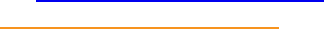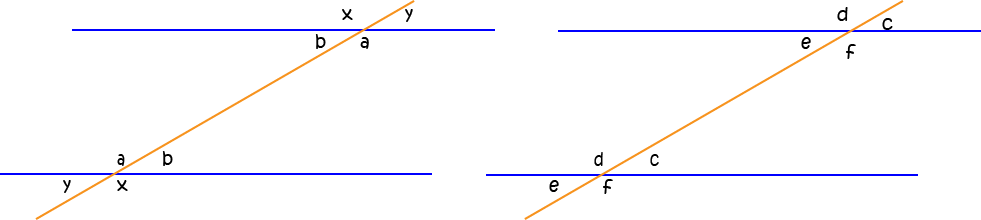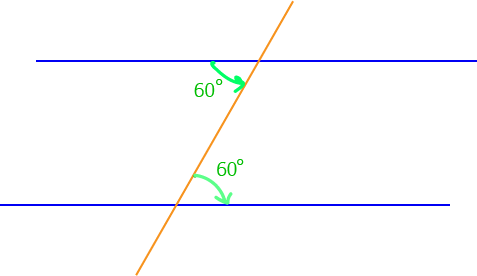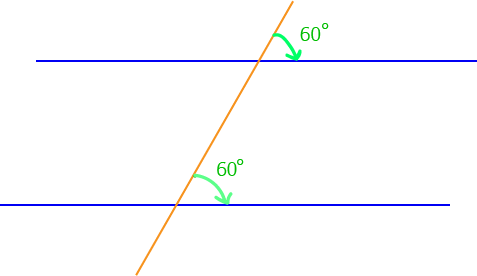# Parallel Lines and Pairs of AnglesTwo lines are parallel if they always stay the same distance apart and never cross each other.

In the examples below, the orange and blue lines are parallelExample 1Example 2

## Pairs of Angles and Parallel Lines

A line that crosses two or more other lines is called a transversal. When a pair of parallel lines is crossed by a transversal, a number of different pairs of angles are formed, many of which are equal, while others are supplementary (add to $180^\circ$). The diagrams below show these different angles.In the picture on the left, the blue lines are parallel and the orange line is a transversal. In addition:

• The pairs of angles labelled by $a$ and $b$ are alternate angles
• The pairs of angles labelled by $x$ and $y$ are alternate exterior angles
As the blue lines are parallel, each pair of alternate angles is equal and each pair of alternate exterior angles is equal.

In the picture on the right, the blue lines are parallel and the orange line is a transversal. In addition:

• The pairs of angles labelled by $c$, $d$, $e$ and $f$ are corresponding angles
• The pairs of angles labelled by $c$ and $f$, and $d$ and $e$ are cointerior angles
As the blue lines are parallel, each pair of corresponding angles is equal and each pair of cointerior angles is supplementary (adds up to $180^\circ$).

## Tests for Parallel Lines

Each type of angle pair gives rise to a test for parallel lines:

1. If the two angles in any pair of alternate angles formed when a transversal cuts a pair of lines are equal, then the pair of lines is parallel
2. If the two angles in any pair of corresponding angles formed when a transversal cuts a pair of lines are equal, then the pair of lines is parallel
3. If the two angles in any pair of alternate exterior angles formed when a transversal cuts a pair of lines are equal, then the pair of lines is parallel
4. If the two angles in any pair of cointerior angles formed when a transversal cuts a pair of lines are supplementary, then the pair of lines is parallel

So, if you have two lines cut by a transversal and you find a pair of alternate angles that are not equal, or a pair of alternate exterior angles that are not equal, or a pair of corresponding lines that are not equal, or a pair of cointerior angles that are not supplementary, then you know that the two lines are not parallel.

Let's look at some examples.

### ExamplesIn the picture, both alternate angles marked in green are equal to $60^\circ$. So, the two blue lines are parallel.In the picture, one of the alternate angles is equal to $124^\circ$, but the other is equal to $122^\circ$. The two blue lines are not parallel.The picture shows a pair of corresponding angles that are equal (to $60^\circ$). Therefore, the two blue lines are parallel.In the picture, one of the corresponding angles marked is $124^\circ$, while the other is $122^\circ$. As these corresponding angles are unequal, the two blue lines are not parallel.The picture shows a pair of cointerior angles that is supplementary ($120^\circ + 60^\circ = 180^\circ$). Therefore, the two blue lines are parallel.In the picture, the pair of cointerior angles adds up to $57^\circ + 124^\circ = 181^\circ \neq 180^\circ$. As this pair of cointerior angles is not supplementary, the two blue lines are not parallel.

### Description

In this mini book, you will learn about

1. Alternate angles
2. Cointerior angles
4. Vertically Opposite Angles

and several other topics related to lines and angles.

### Audience

Year 10 or higher

### Learning Objectives

To learn the basics of Lines and Angles stream of Geometry

Author: Subject Coach
You must be logged in as Student to ask a Question.